# Identify the levels of your hierarchy as preparation for special calculations

If you have a multi-level hierarchy in TARGIT, you can refer to levels with modifiers that can help you refer to:

• Children (the level below the current one)
• Siblings (all dimension values one the same level as the current one)
• Levels (level 0 being the all level of a dimension, level 1 being the top of the hierarchy, level 2 next level etc)

However, it is difficult in certain scenario to identify which level you are currently addressing.

Let's say you want to create a column that does different calculations on different levels of the hierarchy.
Then you would like to write something like:

if level1 then calculation1 else if level2 then calculation2 else if level3 then calculation3 etc...

That's not possible out of the box, but here's a few intermediate calculations that allows you to do just that.

Here's a crosstab showing a hierarchy with 4 levels: Terrritory, Country, Region and Customer: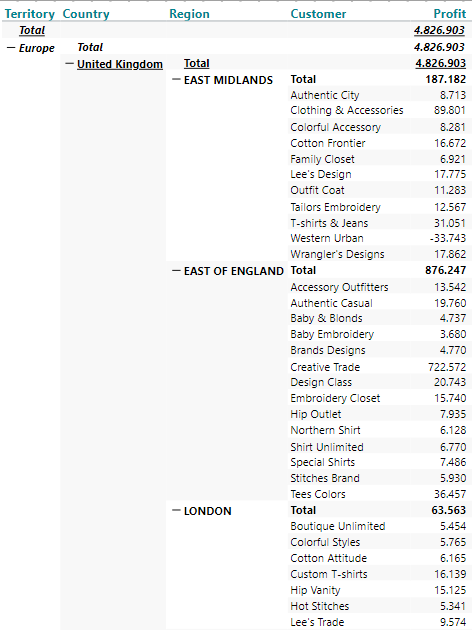Now we start from the bottom to identify levels.

1st calculation (as a new measure) uses this syntax to identify lowest level (level 4 in this case) of the hierarchy:
if allcount(d1, all(c), m1) = 0 then 4 else 0

If a row has no "children", we are at the lowest level of the hierarchy, and we will mark these up with the value 4.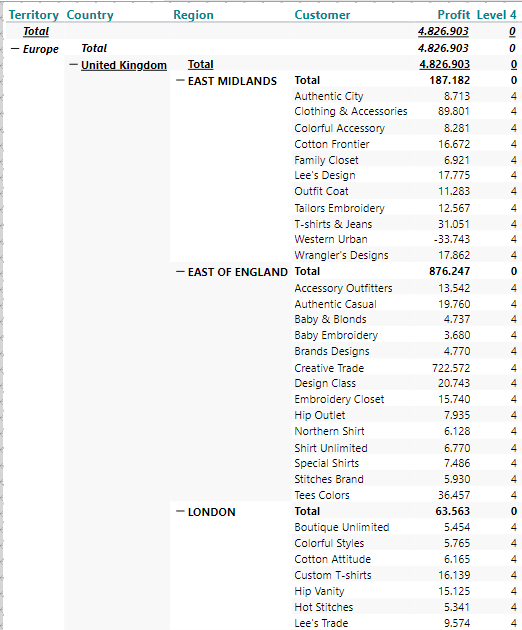Now we have a new measure (m2). We can use this to move up to next level of the hierarchy with this calculation (as a new measure):

if max(d1, all(c), m2) = 4 and min(d1, all(c), m2) = 4 then 3 else 0

This does a test on measure 2 (m2) - which is my previous calculation and say if both min and max are 4 - I'm pretty sure that we are looking at the rows identified in the previous calculation.

If these rows are the children of the current level - then that level must be the one above level 4.

Result: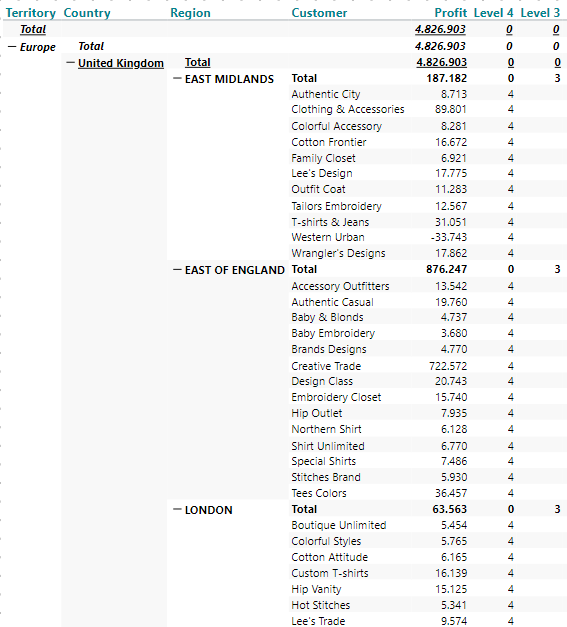Same method applied on the next level with this syntax:

if max(d1, all(c), m3) = 3 and min(d1, all(c), m3) = 3 then 2 else 0

And one more time with this calculation:

if max(d1, all(c), m4) = 2 and min(d1, all(c), m4) = 2 then 1 else 0

Now we have this intermediate result: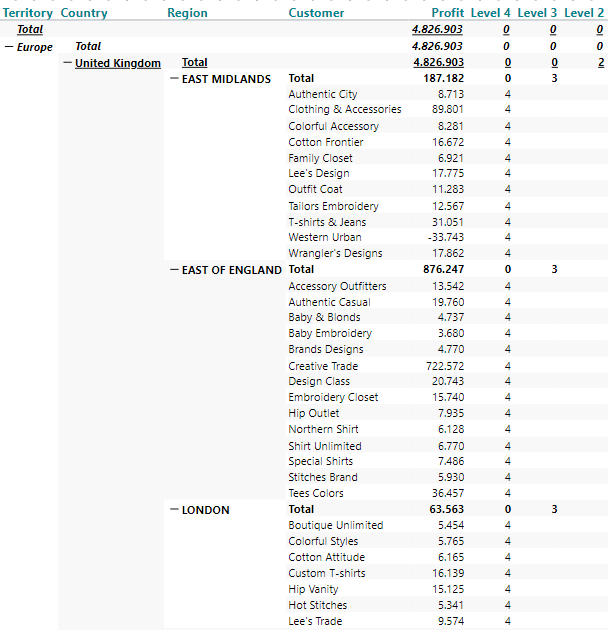Our final calculation just sums op measures 2 to 5 (our 4 calculations):

sum(all, 0, m2:m5)

Now we are there: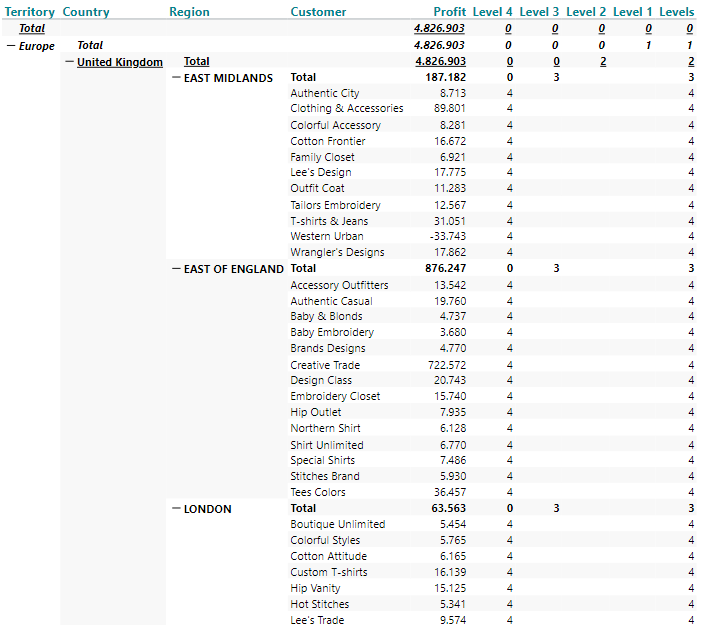All this was just prerequisites to be able to write syntax like this:

if sum(d1,0,m6)= 1 then calculation1 else if sum(d1,0,m6)=2 then calculation2 else if sum(d1,0,m6)=3 then calculation3 else calculation4

NOTE: This tip works when your hierarchy is fully expanded. With a partially expanded hierarchy, you will get results that might not be what you expected.

0 out of 0 found this helpful

• Hi Niels

Thanks for the trick.

It works perfectly when I have expanded the hierarchy: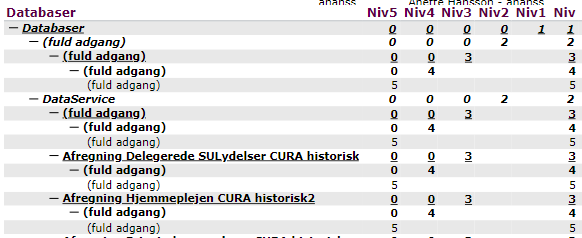But when I collapse the hierarchy or use the filter, the first levels disappear.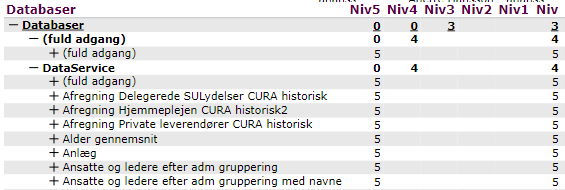Is it possible to insert a condition to test how many levels it open? Fx a formel that tests the level of the first row in the hierarchy (the value of "Niv" in the first row)? To modify the number of levels.

• Got it - Just added +1-sum(all,0l(1),m2:m5) to the calculation :)